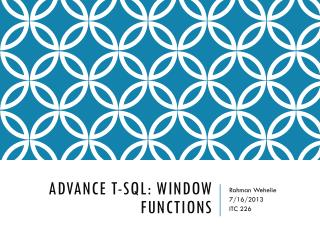Download Presentation# Advance T-SQL: Window Functions - PowerPoint PPT Presentation

Download PresentationDownload Policy: Content on the Website is provided to you AS IS for your information and personal use and may not be sold / licensed / shared on other websites without getting consent from its author. While downloading, if for some reason you are not able to download a presentation, the publisher may have deleted the file from their server.

- - - - - - - - - - - - - - - - - - - - - - - - - - - E N D - - - - - - - - - - - - - - - - - - - - - - - - - - -
##### Presentation Transcript

1. Advance T-SQL: Window Functions Rahman Wehelie 7/16/2013 ITC 226

2. Windowing • Window Function belong to a type of function called 'set function‘ • Window is used to refer to set of rows that the function works on • Window were added to SQL:2003 - the fifth revision of the SQL database query language. • other DBMSs such as Oracle, Sybase and DB2 have had support for window functions • SQL Server has had only a partial implementation until SQL 2012 • You implement window functions as part of a query’s SELECT expression

3. Main Benefit • A big benefits of window functions is that we can access the detail of the rows from an aggregation • GOCREATE TABLE Table (ID INT, Value Numeric(18,2))GOINSERT INTO Table (ID, Value) VALUES(1, 50.3), (1, 123.3), (1, 132.9),      (2, 50.3), (2, 123.3), (2, 132.9), (2, 88.9),      (3, 50.3), (3, 123.3);GO

4. Aggregation • If we sum the value column by conventional GROUP By • SELECT ID, SUM(Value)  FROM TableGROUP BY ID;

5. Cont… • Here is the set that SUM aggregation function worked on • Because we applied the aggregation function in the column value, grouping the results by ID, we the lose the details of the data

6. Row Details • Suppose you need to write a query to return the total /average/quantity value of sales for each ID, and still return the actual values of the rows • SELECT ID,       Value,       SUM(Value) AS "Sum"       AVG(Value) AS "Avg"       COUNT(Value) AS "Quantity"   FROM TableGROUP BY ID; • Column 'Table.Value' is invalid in the select list because it is not contained in either an aggregate function or the GROUP BY clause • it is against the way that aggregations work

7. Over() Clause • A commonly used alternative is to write every aggregation into a subquery • The clause OVER() allows us to access the details of the rows that have been aggregated. • SELECT ID,       Value,       SUM(Value) OVER() AS "Sum"       AVG(Value) OVER() AS "Avg"       COUNT(Value) OVER() AS "Quantity"   FROM Table

8. New Window of Data - PARTITION BY • Suppose you want the aggregate the data grouped by ID • To do this, use the clause PARTITION BY clause • SELECT ID,Value,  SUM(Value) OVER(PARTITION BY ID) AS "Sum"AVG(Value) OVER(PARTITION BY ID) AS "Avg"COUNT(Value) OVER(PARTITION BY ID) AS "Quantity"  FROM Table

9. Cont..

10. ROW_NUMBER, RANK, DENSE_RANK and NTILE • To test the functions, let's create a table called Table1 • GOCREATE TABLE Table1 (Col1 INT)GOINSERT INTO Tab1 VALUES(5), (5), (3) , (1)GO

11. Row_Number() • The ROW_NUMBER function is used to generate a sequence of numbers based on a set in a specific order. • -- RowNumberSELECT Col1,        ROW_NUMBER() OVER(ORDER BY Col1 DESC) AS "ROW_NUMBER()"     FROM Table1

12. Rank() & Dense_Rank() • Returns the rank of each row within the partition of a result set. • The rank of a row is one plus the number of ranks that come before the row in question. • Rank() returns the result with a GAP after a tie, whereas the function DENSE_RANK doesn’t.

13. Example • -- RankSELECT Col1,        RANK() OVER(ORDER BY Col1 DESC) AS "RANK()"   FROM Table1GO-- Dense_RankSELECT Col1,        DENSE_RANK() OVER(ORDER BY Col1 DESC) AS "DENSE_RANK"   FROM Table1

14. NTILE() • The NTILE function is used for calculating summary statistics • Distributes the rows in an ordered partition into a specified number of groups. • The groups are numbered, starting at one. For each row, NTILE returns the number of the group to which the row belongs.

15. NTILE Example • -- NTILESELECT Col1,        NTILE(3) OVER(ORDER BY Col1 DESC) AS "NTILE(3)"   FROM Table1 • We can see that 4 rows were divided by 3, the remaining row is added in the initial group • -- NTILESELECT Col1,        NTILE(2) OVER(ORDER BY Col1 DESC) AS "NTILE(2)"  FROM Table1 • In the next example,there are no remained rows